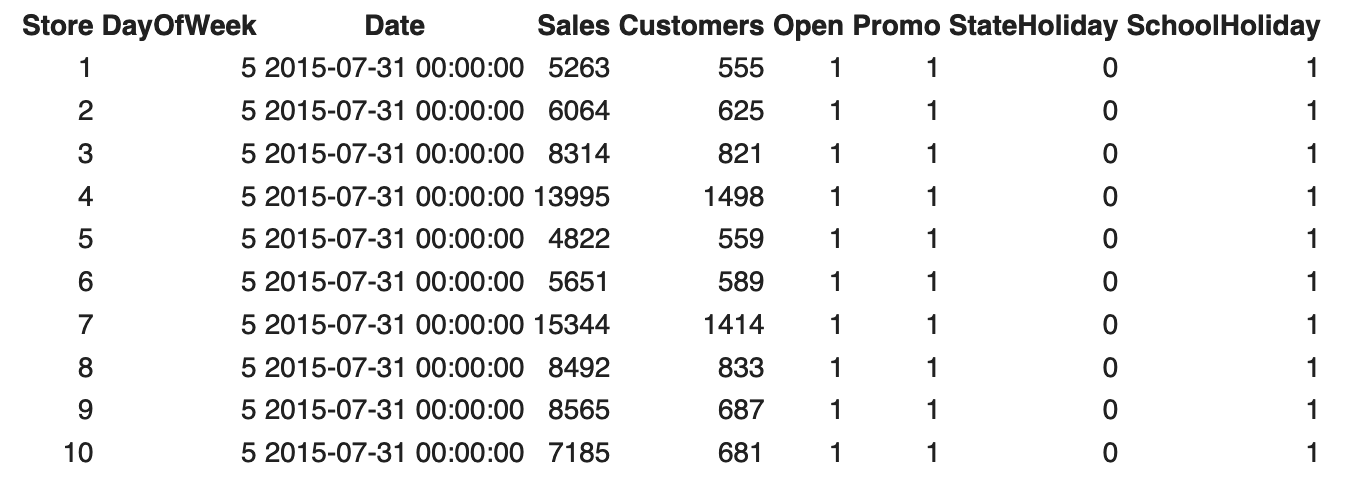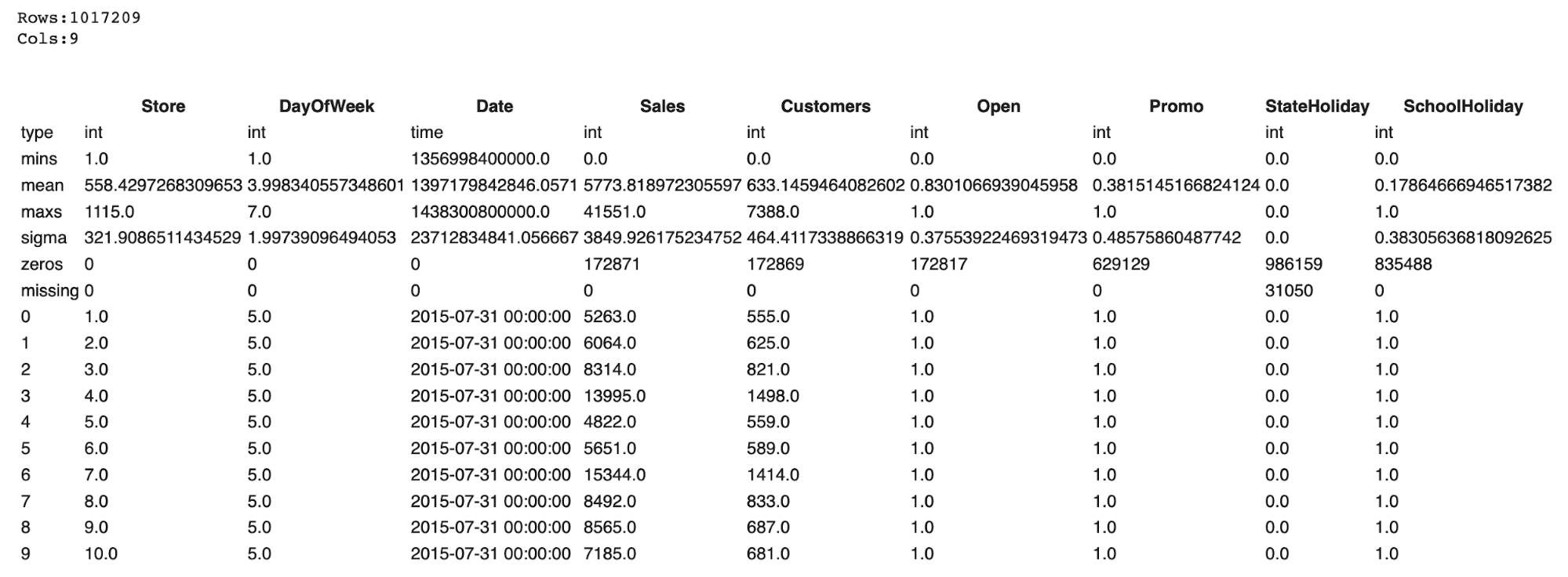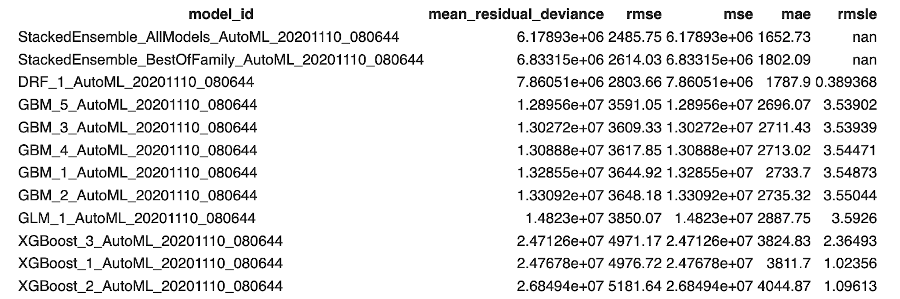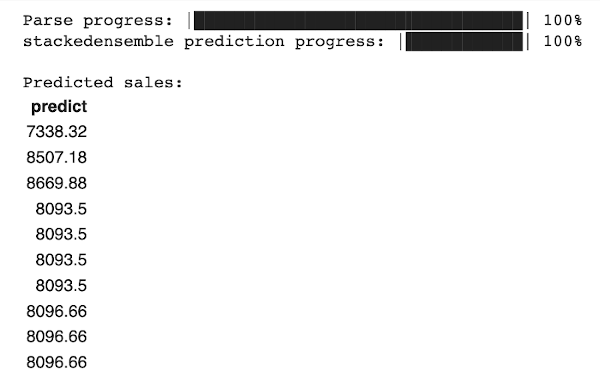| Technical Review: ABCOM Team | Level: intermediate | Banner Image Source : Internet |

# Introduction

Finding the best performing algorithm on a dataset has always been a significant challenge for ML practitioners. There are several libraries available for automatic selection of algorithms; one such platform is H2O that provides an automated comparison and ranking amongst many-many algorithms. Not just this, it also provides for ensembles on different algorithms. Not just the algorithms, but it also does evaluation on different DNN (Deep Neural Networks.) In this short tutorial, I will show you how to use H2O for selecting the best performing model for your dataset and also on a custom DNN.

# What is AutoML?

Machine learning is the most talked about technology in today’s world. However, so far this has been the forte of data scientists and machine learning researchers. So, what about the new comers in this field? Do they have to learn all the fundamentals of machine learning to apply machine learning in their real-world applications? A machine learning practitioner applies various machine learning algorithms on his pre-processed dataset, fine-tuning the various hyper-parameters used by the algorithm and eventually developing and selecting the best algorithm that gives the best prediction. This entire process is now automated, and that’s what AutoML is all about. There are many AutoML solutions available in this world; H2O is one of them. The others, just to mention a few, are Auto-sklearn and Google’s Cloud AutoML. In this tutorial, I will show you how to use the AutoML solution of the H2O framework.

# What is H2O?

H2O is an open source machine learning platform for machine learning. If you want a quick introduction to H2O, check our video tutorial, H2O - a Quick Start. We will use H2O AutoML to test about 30 different algorithms on our dataset and rank them using their performance.

# Case Study for AutoML

To show the use of AutoML, I will use a sales prediction dataset provided by Kaggle. The task is to predict the future sales using the features provided in the data. They collected the data over 3,000 drug stores in 7 European countries. Several factors such as promotions, competition, school and state holidays affect the sales; these parameters would become the features for our ML model training. They provide the data in CSV format. Just for your information, additionally H2O supports the following file types:

• SVMLight
• ARFF
• XLS (BIFF 8 only)
• XLSX (BIFF 8 only)
• Avro version 1.8.0 (without multi file parsing or column type modification)
• Parquet

## Creating Project

Create a new Google Colab project and rename it to AutoML-H2O. If you are new to Colab, then check out this short video tutorial on Google Colab.
You will need to install H2O in your Colab environment, do so using following command:

``````pip install h2o
``````

Import H2O python module and H2OAutoML class and initialize a local H2O cluster. Every new Python session begins by initializing a connection between the python client and the H2O cluster.

``````import h2o
from h2o.automl import H2OAutoML
h2o.init()
``````

``````!wget https://github.com/abcom-mltutorials/AutoML-H2O/blob/main/rossmann-store-sales.zip?raw=true
``````

Unzip the dataset for further processing. It contains the following four CSV files.

• sample_submission.csv
• store.csv
• test.csv
• train.csv

We will use train.csv for training our model and test.csv for testing the model’s performance.
Load the CSV file containing the dataset into the project and examine its few records.

``````traindf= h2o.import_file("/content/train.csv")
``````

The output is as follows:You may check the data types and the number of data points using the describe method:

``````traindf.describe()
``````

The partial output is shown below:As you can see, it has whooping 1 million plus data points and totally nine columns.

Since we are trying to predict the future sales, the Sales column becomes our target. To keep it simple, I am going to use the rest of the columns as features. Ideally, a data scientist having a domain knowledge will select the most appropriate fields as features for model development.

We set the features and target in the following two statements:

``````features=['Store','DayOfWeek','Date','Open','Promo','StateHoliday','SchoolHoliday']
target='Sales'
``````

We now look at what ML algorithms they use for comparisons in AutoML?

## List of Models in AutoML

The current version of AutoML trains and cross-validates the following algorithms, in the following order:

• Three pre-specified XGBoost GBM (Gradient Boosting Machine) models,
• Fixed grid of GLMs,
• Default Random Forest (DRF),
• Five pre-specified H2O GBMs,
• Near-default Deep Neural Net,
• Extremely Randomized Forest (XRT),
• Random grid of XGBoost GBMs,
• Random grid of H2O GBMs, and
• Random grid of Deep Neural Nets.

In some situations, the evaluation process may talk longer than the user-set time limit, in this case, the results of a few algorithms would miss in the leaderboard. The leaderboard also contains two Stacked Ensemble models; I will explain this process in a later section. Optionally, you may switch off particular algorithms (or groups of algorithms) using the exclude_algos argument.

The code below shows you how to use AutoML.

``````aml= H2OAutoML(max_models=10,max_runtime_secs=300,seed=1)
aml.train(x=features,y=target,training_frame=traindf)
``````

The `max_models` determines the number of models you want to train on. The `max_runtime_secs` argument provides a way to limit the training time.
The output is a leaderboard providing the ranking for all tested algorithms. We generate the leaderboard using following statement:

``````result = h2o.automl.get_leaderboard(aml, extra_columns = 'ALL')
``````

The output is as follows:In the screenshot above, you see the ten top-ranked models along with their validation metrics. In our case, the best performing model is the Stacked Ensemble.

## What is Ensemble?

In ensemble learning, we strategically generate multiple models and combine their predictions. In H2O, it is a supervised learning algorithm that uses stacking to find the optimal combination on a collection of algorithms. We also call the stacking super learning. In stacked learning or the regression as it is, a second-level “meta learner” is used to find the optimal combination of the base learners. The goal is to ensemble strong, diverse sets of learners together. This is unlike bagging and boosting techniques that you might have learned in tree-based algorithms.

## Model Inference

The aml variable contains the best performing model. You use the predict method on this instance to do the model inference. We will do so on our test data:

``````testdf= h2o.import_file('/content/test.csv')
prediction = aml.predict(testdf)
print ("\nPredicted sales: ", prediction)
``````

The output is as follows:The output shows the sales prediction for each data point (region) in the test data.

Having seen the selection amongst the statistical models, I will now show you H2O’s capability of fine tuning a tailor-made deep neural network.

## H2O on DNN

H2O provides an Estimator for building and testing deep neural networks. Estimators provide a model-level abstraction and encapsulates several stages of ML development to do a quick development and testing. Import the deep learning estimator using the following statement:

``````from h2o.estimators import H2ODeepLearningEstimator
``````

Import the training dataset and split it into training and testing:

``````df= h2o.import_file("/content/train.csv")
train, test = df.split_frame([0.8], seed=42)
``````

Next, you build an estimator based model using following statement:

``````dl = H2ODeepLearningEstimator(distribution="tweedie",
hidden=,
epochs=1000,
train_samples_per_iteration=-1,
input_dropout_ratio=0.1,
activation="Tanh",
single_node_mode=False,
score_training_samples=0,
score_validation_samples=0
)
``````

Here is a description of the various parameters:

• Distribution - this is the loss function and can take any of the following values
• AUTO
• Beernoulli
• Multinomial
• Gaussian
• Poisson
• Gamma
• Laplace
• Quantile
• Huber
• Tweedie

Though a detailed explanation of these loss functions is beyond the scope of this tutorial; it may suffice to say here that bernoulli and multinomial are used for binomial and categorical types of outputs, while we use the rest for numeric.

• Hidden - Specifies number of hidden layers in the network
• epochs - Number of epochs
• train_samples_per_iteration - Specifies number of training samples used on each epoch
• input_dropout_ratio - Used for generalization.
• activation - The permissible values are:
• Tanh
• Tanh with dropout
• Rectifier
• Rectifier with dropout
• Maxout
• Maxout with dropout.
• single_node_mode - Used for fine-tuning of model parameters.
• score_training_samples - Specifies number of training samples (split) for scoring
• score_validation_samples - Specify the number of validation samples (split) for scoring

Like earlier case, we set features and target:

``````features=['Store','DayOfWeek','Date','Open','Promo','StateHoliday','SchoolHoliday']
target='Sales'
``````

We train the model by calling the train method on the estimator:

``````dl.train(x=features,y=target,training_frame=train,validation_frame=test)
We evaluate the performance using model_performance:
dlperformance = dl.model_performance()
dlperformance
``````

The partial output is as shown below:

``````ModelMetricsRegression: deeplearning
** Reported on train data. **

MSE: 6857417.442711691
RMSE: 2618.6671118551308
MAE: 1729.311314647968
RMSLE: 0.36796968993556445
Mean Residual Deviance: 10.558378472342577
``````

Once you are satisfied with the performance, use the model's predict method to do predictions on any unseen data.

# Summary

AutoML eases the life of ML practitioners in choosing the best performing model on their datasets. In this tutorial, you learned how to use the AutoML feature of H2O for model selection. H2O also provides a feature for developing a DNN based model development with fine-tuning its hyper-parameters. In this tutorial, I have shown this capability of DNN development using H2O. There are other AutoML tools available in the market, I find this trivial to use and efficient.